×#### Thank you for registering.

One of our academic counsellors will contact you within 1 working day.

Click to Chat

1800-1023-196

+91-120-4616500

CART 0

• 0

MY CART (5)

Use Coupon: CART20 and get 20% off on all online Study Material

ITEM
DETAILS
MRP
DISCOUNT
FINAL PRICE
Total Price: Rs.

There are no items in this cart.
Continue Shopping```Solved Examples of Definite Integral Part I11:     The area bounded by the curve y = x (3 – x)2 , the x – axis and the ordinates of the maximum and minimum points of the curve is

(A)       2 sq. units

(B)       4 sq. units

(C)       3 sq. units

(D)       8 sq. units

Solution:             y = x (3 – x)2

After solving , we get x =1 and x = 3         are       points of maximum and     minimum respectively.

Now the shaded region is the required region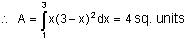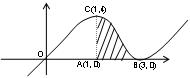12:                        The area enclosed by the curve |x + 1| + |y + 1| = 2 is

(A)       3 sq. units                       (B)       4 sq. units

(C)       5 sq. units                       (D)       8 sq. units

Solution:             Shift the origin at the point (-1, -1)

So |x + 1| + |y + 1| = 2

becomes |x| + | y | = 2

Hence required area is

= 4 × 1/2 × 2 × 2 = 8sq. units13:                             The area common to the curves y = x3 and y =  √x is

(A)       2                                        (B)       4

(C)       8                                        (D)       None of these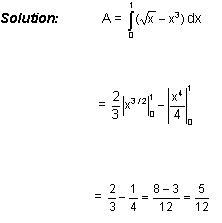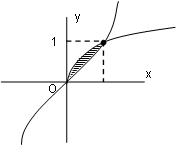14:       The area of the region consisting of points (x, y) satisfying |x ± y | < 2 and x2 + y2 > 2 is

(A)             8 – 2π sq. units

(B)             4 – 2π sq. units

(C)             1 – 2π sq. units

(D)             2π sq. units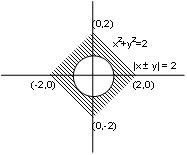Solution:            Shaded region is the required one.

Required Area   =  4 × 1/2 × 2 × 2 – π.2 = 8 – 2π sq. unit

15:                   ∫xo [sint] dt where x ∈ and [2nπ, (4n+1)]π, n ∈ N and [.] denotes the greatest integer function is equal to.

(A)       -nπ                                         (B)       -(n +1)π

(C)       -2nπ                                      (D)       -2(n +1)π16:                  If f(π) = 2 and ∫πo [f(x) + f"(x)] sin x dx = 5 then f(0) is equal to, (it given that f(x) is continuous in [0, π])

(A)       7                      (B)       3                      (C)       5                      (D)       1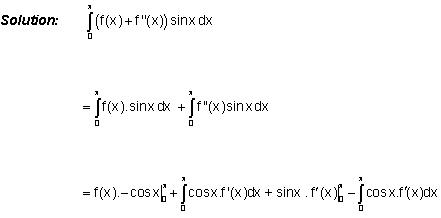=> f(π) + f(0) = 5 (given)

=> f(0) = 5 – f(π) = 5 – 2 = 3

17:                Let f(x) is a continuous function for all real values of x and satisfies+ a then value of ‘a’ is equal to

(A)  –1/24                   (B)      17/168                (C)      1/7        (D)      None of theseDiff. both sides of (i) w. r. t. x we get;

f(x) = 0 – x2 f(x) + 2x15 + 2x5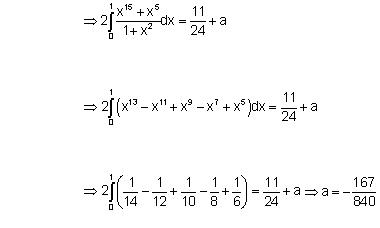18: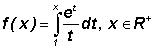. Then complete set of values of x for which f(x) <lnx is

(A)       (0, 1]                                       (B)       [1, ∞)

(C)       (0, ∞)                                      (D)       None of these

Solution: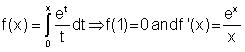Let g(x) = f(x) - ln(x). x ∈ R +

=> g ’(x) = f ’(x) – 1/x = ex – 1 / x > 0 ∀ x ∈ R+

=> g'(x) is increasing for ∀ x ∈ R+

g(1) = f(1) - ln1 = 0 – 0 = 0

=> g(x) > 0 ∀ x > 1 and g(x) > 0      ∀ x ∈ (0, 1]

=> ln x > f(x) ∀ x ∈ (0, 1]

19: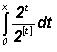, where [.] denotes the greatest integer function, and x ∈ R+, is equal to

(A)       1/In2 ([x] + 2[x]–1)            (B)       1/In2 ([x] + 2[x])

(C)       1/In2 ([x] – 2[x])               (D)       1/In2 ([x] + 2[x]+1)

Solution:       Let n < x < n + 1 where n ∈ I, I > 0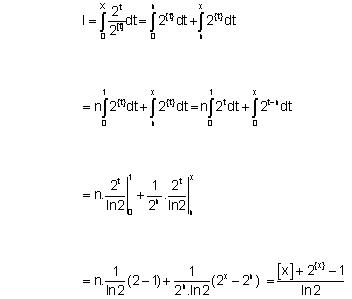20:                  Let I1 =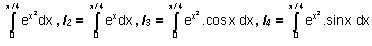then

(A)      I1 > I2 > I3 > I4                         (B)      I2 > I3 > I4 > I1

(C)      I3 > I4 > I1 > I2                         (D)      I2 > I1 > I3 > I4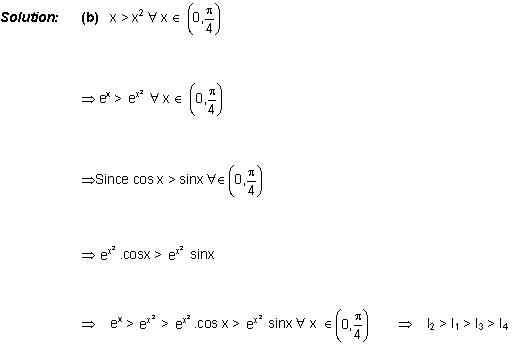To read more, Buy study materials of Definite integral comprising study notes, revision notes, video lectures, previous year solved questions etc. Also browse for more study materials on Mathematics here.
```### Course Features

• 731 Video Lectures
• Revision Notes
• Previous Year Papers
• Mind Map
• Study Planner
• NCERT Solutions
• Discussion Forum
• Test paper with Video Solution